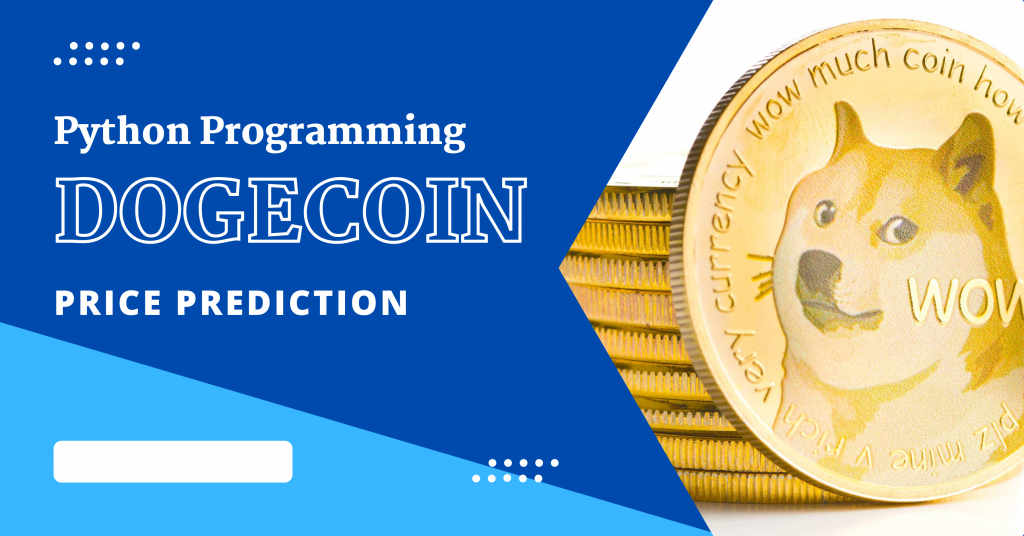# Crypto Price Prediction with PythonToday in this tutorial, we are going to use a machine-learning algorithm to predict the future prices of a cryptocurrency known as Dogecoin. We are making use of Python as a programming language.

## What is Dogecoin?

`Dogecoin` is a cryptocurrency with a pleasant brand image that seeks to be a helpful introduction to cryptocurrencies. Dogecoin, also known as the “joke” coin, was proposed by Billy Markus, an Oregon programmer.

He reasoned that a less serious coin, such as Dogecoin, would be more likely to be accepted by the general public than Bitcoin even with less scale.

Dogecoin may be used to make payments and buy things, but it’s not a great way to hold value. This is mostly due to the fact that Dogecoin has no lifespan limit on the number of coins that may be generated through mining, making it inherently extremely inflationary.

## 1. Importing Modules

Along with importing various modules such as numpy, pandas, matplotlib, and seaborn, we also set the plotting style and set the seaborn plot as well.

```import numpy as np
import pandas as pd
import matplotlib.pyplot as plt
import seaborn as sns
from seaborn import regression
sns.set()
plt.style.use('seaborn-whitegrid')
```

## 2. Explore the Data

The dataset contains over 2.5k datapoints and 7 attributes namely the opening and closing prices on various days.

```data = pd.read_csv("Dogecoin.csv")
print("Shape of Dataset is: ",data.shape,"\n")
```

## 3. Visualize the Dogecoin Data

No ML model is complete without visualizing the dataset using the matplotlib library which can be achieved using the code mentioned below.

We would be visualizing the `Close` attribute along with `Date` attribute using the linear line plot.

```data.dropna()
plt.figure(figsize=(10, 4))
plt.title("DogeCoin Price INR")
plt.xlabel("Date")
plt.ylabel("Close")
plt.plot(data["Close"])
plt.show()
```

## 4. Applying Machine Learning Model

As the ML model, for the Dogecoin dataset, we will be using the `AutoTS` model and import the model into the program.

Then, create an AutoTS model object in order to fit the data points into the model using the fit function and then predict the prices for all data points using the `predict` function.

In the end, display the prices predicted by the AutoTS model. The code to obtain the aim is mentioned below.

```from autots import AutoTS
model = AutoTS(forecast_length=10, frequency='infer', ensemble='simple', drop_data_older_than_periods=200)
model = model.fit(data, date_col='Date', value_col='Close', id_col=None)

prediction = model.predict()
forecast = prediction.forecast
print("DogeCoin Price Prediction")
print(forecast)
```